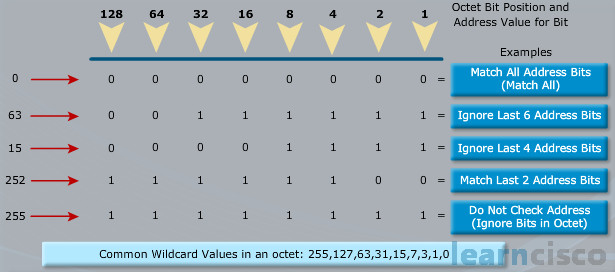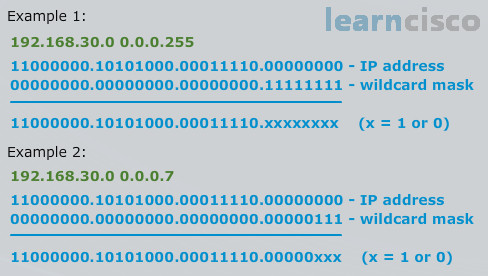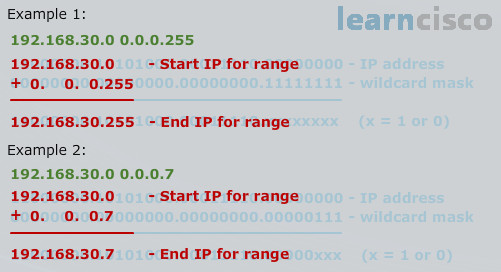ICND1 100-105

# Access Control Lists Wildcard Masking

We will spend some time later on digging into the syntax of access control lists and there are many parts and components that make up the syntax. But one of those parts is known as the wildcard mask and it goes hand-in-hand with an address. And we have to have a solid understanding of the wildcard mask. If we don't, it makes it difficult to craft access control lists and it makes it difficult to understand what the access control list is trying to accomplish. Let's think about this word wildcard mask. Well in your IT career, have you experienced other wildcards? What about in the Microsoft command line or Microsoft DOS? There are some wildcard symbols in that. Something that comes to mind is the asterix. Yeah that would be, you might do it like a copy *.* right? And even the question mark symbol is a form of wildcard mask. So, we are familiar with wildcards in general as human beings and hopefully, IT career path people as well.

If I got a zero in the position of the wildcard mask, that means match that IP address bit exactly. All right, if I have a one in the wildcard mask position, that means the IP address bit could be either a one or a zero because those are the only two options with binary, one or zero, which really means ignore whatever is there. If it's a one, no big deal, it could be a zero, but zero could be a one. We'll see examples of these. Down below, what are the common wildcard values we might find in an octet when we were dealing with a wildcard mask?Okay, so we have been talking about the bitwise operation of this wildcard mask that we are using, but the reality is when we input them, we are not inputting them as bits, we are inputting them as dotted decimal values and so we see the 255 and 127 and 63. Now we mentioned contiguous bits and in all of those cases, we have contiguous ones, but we can see a few examples of those bottom values. We see the 63, that's the second row. Look at that, we do have contiguous ones. We should be expecting contiguous ones, but where our subnet mask would have contiguous ones on the left, now our contiguous ones are on the right-hand side.

And that's something to be very careful with. The 252, look at that fourth row, that fourth example in the chart. We should never input something like that, never input something like that in the parameter field where a wildcard mask is expected. Why? Because our access control list don't want subnet mask octet values. Think about like 128, 192, 224, 240, 248, 252, that's one of them right there, 254, all of those should not be found inside of our typical access control list wildcard mask. Why? Because we are reversing the ones and zeros, which is going to mean, our access control list is going to be behaving wildly different from what we want.

Okay, so if you were to ever see an access control list that was constructed with something that looks more like a subnet mask than a wildcard mask, that's a warning sign to you. Alarms should be going off and you should be looking at that. Okay, so what we are doing in those cases though. Let's look at the 63 example, that's the second row here and one of the wildcard mask example that is down below as well. That means we are wildcarding out the latter six bits. So we are looking at the first two bits, and then wildcarding out everything else and we might use this to correspond to a subnet. We might have like a /26 subnet and so we would use a 63 to match the entirety of that subnet.

Now we are going to teach you how to figure that out because you are absolutely responsible for mastery of the wildcard mask in this discussion.

In order to master the wildcard mask, you have to understand what is happening deep down inside. And the best way to do that is to review it in binary, but let's pause for a moment and remind ourselves of our subnetting discussion. Do we enjoy working with binary? Remember what we mentioned way back? No we don't, we don't. We despise working with binary, so this is not how we want to do it, but this shows you the underlying fundamentals of what is happening. Look at our address in example one, 192.168.30.0 with a wildcard mask of 0.0.0.255. Here we can really see now with that binary analysis, what we are talking about when we are wildcarding out something.Which octet are we wildcarding in example one? That's my question to you readers out there. Well it looks to me that we are wildcarding out the very last octet in this example. Okay in example two, we have another good example, but this one is a lot harder. Oh man, look at that, in example two, we are wildcarding out the last three binary values in the fourth octet. All right, remember how we said zeros mean match, ones mean ignore. Look at that from a binary perspective here. For example 1, everywhere you see a zero in the wildcard mask, look how we have to match the IP address bit perfectly. See that, if you look underneath the dotted line at the solution of comparing the IP address to the wildcard mask, 11000... it's a perfect match, it's a perfect match with the IP address. But that last octet we wildcarded it out, we have xs, xs mean it can be a one or zero. If every single bit in that last octet was a zero, what would the IP address be? Zero. So we would have 192.168.30.0. What if every bit was a one? Then it would be the maximum value of an octet, which would be 255. So 192.168.30.255, there would be no other possible values larger than that or lower than our first value. So with an address and a wildcard mask combination, I have just defined a range of addresses, haven't I? I have just defined 192.168.30.0 through 192.168.30.255 with that combination.

That's really the punch line here. Did you catch it? This is where we are going to go well beyond and teach you things that are not known to many mere mortals and that is how to truly analyze the wildcard mask as a range and with this set of techniques, you are going to be extraordinarily empowered, so pay real attention here. If you still don't grasp the range concept, look at example two, those last three bits are xs, it could be one or zero. If they are all zeros, then what would be the address? 192.168.30.0.

But if they are all ones in that final octet, those last three bits, what would the maximum possible value of that final octet be? Well if I'm doing it from binary analysis, which I don't want to do it this way, it would be one plus two plus four which equals seven. Okay, how did I get that? Well look at the last three ones, the only three ones of the wildcard mask as an example two and that's the significance. One, two, and four, that equals seven. So it sounds to me like we're implying that the example two would match in the fourth octet from 0 to 7.

That's right, so we have a range, an address range from 192.168.30.0 through 192.168.30.7 using that address and wildcard mask combination, for example two. All right, so we understand it's a range operator, this wildcard mask. We can be creative for classifying and identifying the traffic for filtering or special marking or circumstances. But we don't have time, especially on an exam to do, what we see here, we have shortcut for you.

We said it's a range operator, so what could we do as a shortcut, so we do not have to worry about binary? Okay, so the first thing I want you to think is, the struggle that we all have when we are reading these. Look at example one, just as the two values, you see the IP address value and then the wildcard mask value and you are being asked to interpret that. Well that's challenging, example two is even more challenging. Well it can be read quite a bit easier than you would expect, we can consider the first value, the IP address portion, the starting IP address. And remember this is a range, so we have a start and end in any range.

The way that we calculate the end is with addition, just raw addition. I know you can add numbers, so what we do is we add the wildcard mask to the IP address, the starting IP address, and the sum of the two is the ending of the range. This works perfectly in all cases, where the wildcard mask is a properly formed contiguous set of ones. It works essentially every time, it works every time. So if I were to do the sum in example one, which is not all that hard, I would add 255 to 0 and the ending for the fourth octet would be a 255.So the starting would be zero, ending would be 255 and that would be the way that we would read it. And example two is harder though, example two is harder, but with this technique, it's as easy as pie. So, how would we do the same technique for example two? With example two, I take the address 192.168.30.0 underneath it, I put the wildcard mask, which is 0.0.0.7. I do raw addition, 192 plus 0 is 192, 168 plus 0 is 168, 30 plus 0 is 30, 0 plus 7 is 7. I have my starting IP, which is 30.0 and I have my ending IP, which is 30.7, there is my range. So you would know that this would match anything from 0 to 7, but it wouldn't be a match for the eighth IP address and the ninth IP address in the fourth octet.

Then I want you to recall what we'd expect to find in a dotted decimal wildcard mask. Do you remember what they were? It's 0, 1, 3, 7, 15, 31, 63, 127 and 255 and there's a pattern there. The pattern is, it's a value one less than a power of two, right. 255 is one less than 256. 127 is one less than 128. So the .7 looks legit, it is, and with this technique, the world is your oyster.